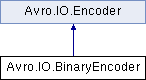Avro C#
Avro.IO.BinaryEncoder Class Reference

Write leaf values. More...

Inheritance diagram for Avro.IO.BinaryEncoder:List of all members.

## Public Member Functions

BinaryEncoder (Stream stream)
void WriteNull ()
null is written as zero bytes
void WriteBoolean (bool b)
true is written as 1 and false 0.
void WriteInt (int value)
int and long values are written using variable-length, zig-zag coding.
void WriteLong (long value)
int and long values are written using variable-length, zig-zag coding.
void WriteFloat (float value)
A float is written as 4 bytes. The float is converted into a 32-bit integer using a method equivalent to Java's floatToIntBits and then encoded in little-endian format.
void WriteDouble (double value)
A double is written as 8 bytes. The double is converted into a 64-bit integer using a method equivalent to Java's doubleToLongBits and then encoded in little-endian format.
void WriteBytes (byte[] value)
Bytes are encoded as a long followed by that many bytes of data.
void WriteString (string value)
A string is encoded as a long followed by that many bytes of UTF-8 encoded character data.
void WriteEnum (int value)
void StartItem ()
void SetItemCount (long value)
void WriteArrayStart ()
void WriteArrayEnd ()
void WriteMapStart ()
void WriteMapEnd ()
void WriteUnionIndex (int value)
void WriteFixed (byte[] data)
void WriteFixed (byte[] data, int start, int len)
void Flush ()

## Detailed Description

Write leaf values.

## Member Function Documentation

 void Avro.IO.BinaryEncoder.WriteBoolean ( bool b ) ` [inline]`

true is written as 1 and false 0.

Parameters:
 b Boolean value to write

Implements Avro.IO.Encoder.

 void Avro.IO.BinaryEncoder.WriteBytes ( byte[] value ) ` [inline]`

Bytes are encoded as a long followed by that many bytes of data.

Parameters:
 value

Implements Avro.IO.Encoder.

 void Avro.IO.BinaryEncoder.WriteDouble ( double value ) ` [inline]`

A double is written as 8 bytes. The double is converted into a 64-bit integer using a method equivalent to Java's doubleToLongBits and then encoded in little-endian format.

Parameters:
 value

Implements Avro.IO.Encoder.

 void Avro.IO.BinaryEncoder.WriteFloat ( float value ) ` [inline]`

A float is written as 4 bytes. The float is converted into a 32-bit integer using a method equivalent to Java's floatToIntBits and then encoded in little-endian format.

Parameters:
 value

Implements Avro.IO.Encoder.

 void Avro.IO.BinaryEncoder.WriteInt ( int value ) ` [inline]`

int and long values are written using variable-length, zig-zag coding.

Parameters:
 datum

Implements Avro.IO.Encoder.

 void Avro.IO.BinaryEncoder.WriteLong ( long value ) ` [inline]`

int and long values are written using variable-length, zig-zag coding.

Parameters:
 datum

Implements Avro.IO.Encoder.

 void Avro.IO.BinaryEncoder.WriteNull ( ) ` [inline]`

null is written as zero bytes

Implements Avro.IO.Encoder.

 void Avro.IO.BinaryEncoder.WriteString ( string value ) ` [inline]`

A string is encoded as a long followed by that many bytes of UTF-8 encoded character data.

Parameters:
 value

Implements Avro.IO.Encoder.

The documentation for this class was generated from the following file:
• src/apache/main/IO/BinaryEncoder.cs